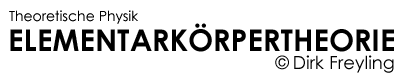Konträr zur Aussage der Standardphysik, die vier Grundkräfte* postuliert, lässt sich Elementarkörper basierend - auf Grund der (auch skalenkorrespondierenden) Masse-Raum-Kopplung - jedwede Wechselwirkung auf das Masse-Radius-Verhältnis der interagierenden Wechselwirkungspartner reduzieren. Das führt zu einer konstruktiven Beschreibungsverarmung, die entweder durch die Radien oder deren reziprok proportionale Massen ausgedrückt werden kann. Mit Hilfe dieser einkomponentigen sprichwörtlichen Minimalbeschreibung lassen sich beispielsweise Grundzustandsenergien, magnetische Momente und insgesamt Materiebildungsmöglichkeiten verstehen und formal analytisch exakt berechnen.

 * Der Begriff Grundkraft ist irreführend, da es sich im Rahmen des Standardmodells der Kosmologie (ΛCDM-Modell) bei der Gravitation um Gleichungssysteme (Einstein, Friedmann) handelt, die in einem mechanischen Sinne keine Kraft beschreiben. Die Schwache und Starke Wechselwirkung sowie die elektromagnetische Wechselwirkung sind gleichfalls erst einmal masselose, "kraftlose", rein mathematische Gebilde.

Ein paar Worte zu den Protagonisten des Für und Wider der Systemphysik

Daß das Gros der thematisch »Wissens-Befähigten« SM-treu und QED-beladen lieber ein neo-barockes Phlogistonmärchen a la Quarks favorisiert und vermeintliche System-Kritiker, u.a. aus Gründen dominierender Egozentrik, leider keinen Blick über ihr selbstgewähltes Tellerrandschicksal wagen, ist zwar menschlich, aber sachlich betrachtet wissenschaftsunwürdig. Viele haben „vergessen“, daß auch berechtigte Kritik (zu den Standardmodellen) nur dann „potent“ ist, wenn voraussagefähige Alternativmodelle wahrgenommen und verbreitet werden. Die Ignoranz vieler alternativer Denker steht der Ignoranz der Systembefürworter in nichts nach.

Der Protonenradius bzw. Modelle die diesen exakt voraussagen sind richtungweisend. Emotionen, unberechtigter Euphemismus, beispielsweise auf Grund fehlender Voraussagefähigkeit, und Mehrheitsdenken gehören nicht zum Wertekanon wissenschaftlicher Betrachtungen. Die Elementarkörpertheorie liefert nicht nur ein konsistentes, minimalistisches, sondern auch ein phänomenologisch begründetes Modell zum Proton, welches eine Masse-Radius-Kopplung aus der Invarianz der (Vakuum-)Lichtgeschwindigkeit zwanglos ableitet.

Die (objekt-)relevanten Einzelergebnisse sind über zielführende Menübegriffe, wie Elementarkörper, Photon, Proton, Elektron, Feinstrukturkonstante,..., Neutrinos,..., Vakuumenergie abrufbar.Sehr bemerkenswert ist die Herleitung der Masse-Energie-Äquivalenz im Rahmen der Masse-Radius-Kopplung. Siehe: Elementarkörper basierende Herleitung der Energie-Masse-Beziehung.

Mathematische Wünsche und Real-Objekt-Wirklichkeiten

Grundsätzlich beginnt das „moderne“ Mißverständnis zur Interpretation eines (quantenmechanischen) Versuches mit der „Idealisierung“, respektive Reduktion, daß der Versuchsaufbau - der additiv Energie in Form von elektrischen oder magnetischen Feldern „zur Verfügung stellt“ – nicht als (energetischer) Wechselwirkungspartner wahrgenommen wird. Werte vermeintlich intrinsischer Objekt-Grössen, wie beispielsweise die »Feinstruktur der Spektrallinien« oder »anomale magnetische Momente« entstehen aber erst durch das „Anlegen“ von äußeren "versuchsinhärenten Feldern“. Dieser logisch nachvollziehbare unvermeidbare „Beobachtungs-Effekt“ wird kategorisch von den Protagonisten der Standardmodell-Physik verdrängt. Es wird so getan, als ob die zusätzliche Energie nur die inneren energetischen Verhältnisse ans „Licht“ bringt, die auch ohne Beobachtung, sprich ohne äußere Energiezufuhr, bereits existieren. Diese Annahme ist nicht nur diskussionswürdig, diese Annahme ist fatal und falsch.

Es drängen sich interdisziplinär Fragen nach der Psychologie der Akteure auf, wie und warum ein (naturwissenschaftlich vor-)gebildeter Mensch plötzlich so unwissenschaftlich falsch agiert? Die ausführliche Beantwortung würde hier den Fokus zu stark verschieben, die kurze Antwort lautet: Bereits die klassische Elektrodynamik wie auch die Quantenelektrodynamik (QED) sind im Kern rein mathematische Konzepte, exemplarisch Divergenz und Unendlichkeit mathematischer Konstrukte rücken in den Vordergrund, phänomenologische Aspekte in den Hintergrund. Real-Objekt-Physik, die sich durch endliche Massen, endliche Ladungen und endliche räumliche Ausdehnungen manifestiert, wird nur insofern thematisiert, wie diese in das angestrebte mathematische Muster passen. Das „Feld“, ob elektrisch, magnetisch oder gravitativ ist aus naturphilosophischer Sicht eine nach wie vor unbekannte sekundäre Grösse, ein sekundärer Begriff. „Feldresultierende Beobachtungen“ lassen sich zwar in Abhängigkeit eines mathematischen Fokus mengenmäßig-ergebnisorientiert u.a. unter Zuhilfenahme virtueller Theorieparameter „nachbauen“, bleiben aber letztendlich ohne plausible Phänomenologie, sozusagen eine Schwarze Box ohne Erkenntnis.

Beispiel: (Anomale) Magnetische Momente

"Ich" sehe was, was "du" nicht siehst …    Die andere Sicht der Dinge

Die experimentellen Penning-Fallen-Ergebnisse für Elektron und Proton - in Form von Frequenzmessungen - in Hinblick auf additive  („anomale“) Beiträge zur semiklassischen Erwartung erscheinen unter dem Aspekt das nicht intrinsische magnetische Momente der Ladungsträger dafür verantwortlich sind, sondern messungsinhärente additive (Magnet-)Feldbeiträge zum magnetischen Moment, die von der jeweiligen Ladungsträgermasse initiiert werden in einem gänzlich anderen Interpretations-Zusammenhang.

Diese additiven Beiträge sind sowohl für Elektron als auch für Proton und unabhängig von der Penning-Falle auch für das Neutron in der Größenordnung von 1·10-26 Joule/Tesla. Der genaue Wert ist abhängig von der Masse des Ladungsträgers im „Feld“, bzw. im Fall des Neutrons von der Gestalt des aus Elektron und Proton gebildeten Neutrons, siehe explizite Berechnungen zum magnetischen Moment des Neutrons.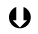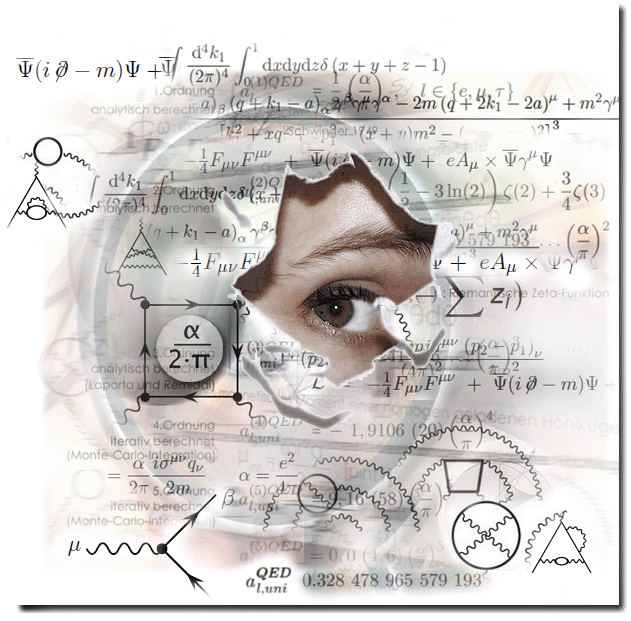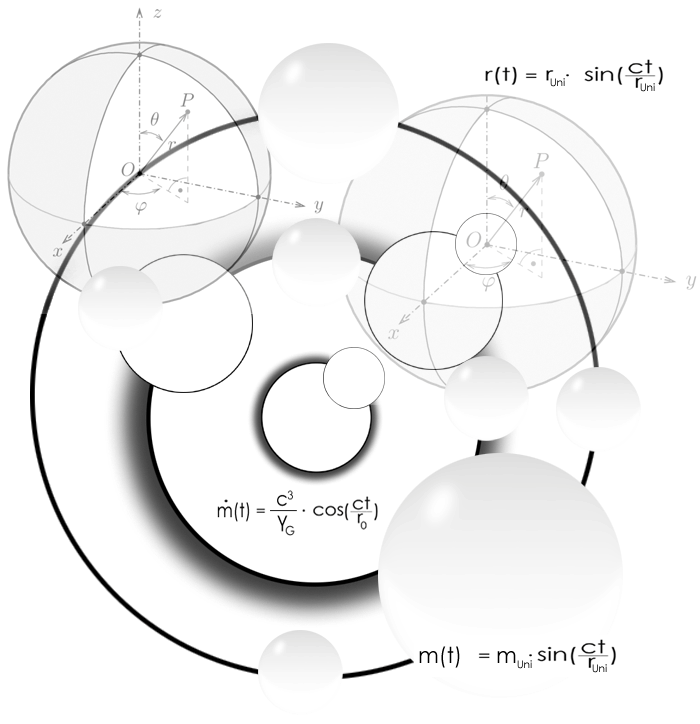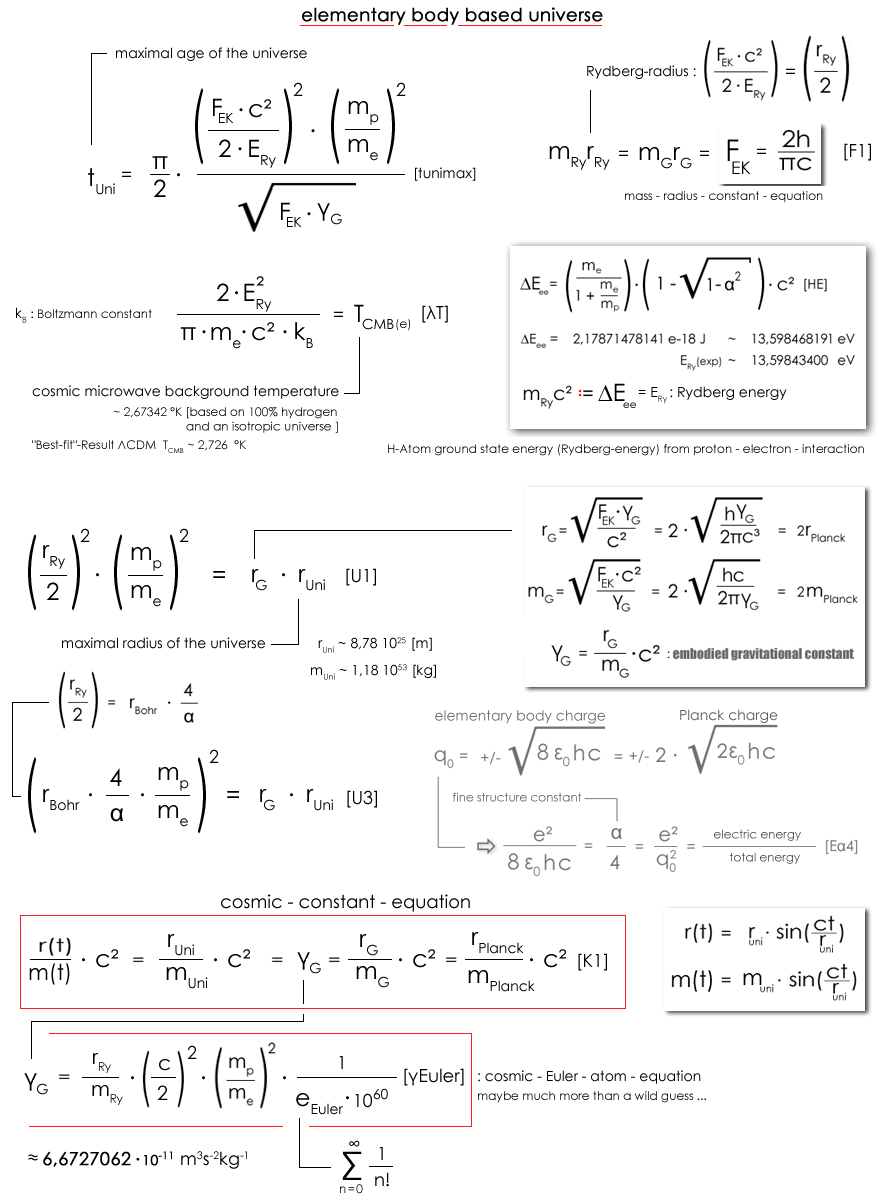Furthermore (in English):

Concept of electric charge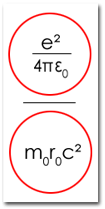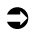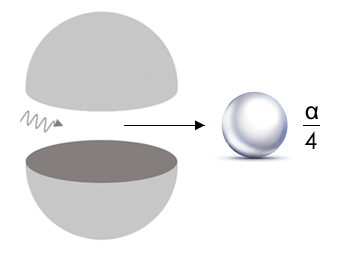Electric charge is a secondary term/concept of standard phycics that suggests a "phenomenological entity" that is uncoupled from the mass (and the radius) of the charge carrier.

Based on elementary-body theory all charge interactions are clearly traceable to mass-radius couplings. Conveniently, electrical charges in the elementary-body model occur only as an implicit function of the Sommerfeld fine-structure constant α as a (formal) result of the mass-radius coupling.

"Keys" for understanding the formation of matter are the phenomenologically founded charge possibilities. First, the energetically (strong) elementary body charge q0 (which energetically equals m0) and the elementary electric charge e.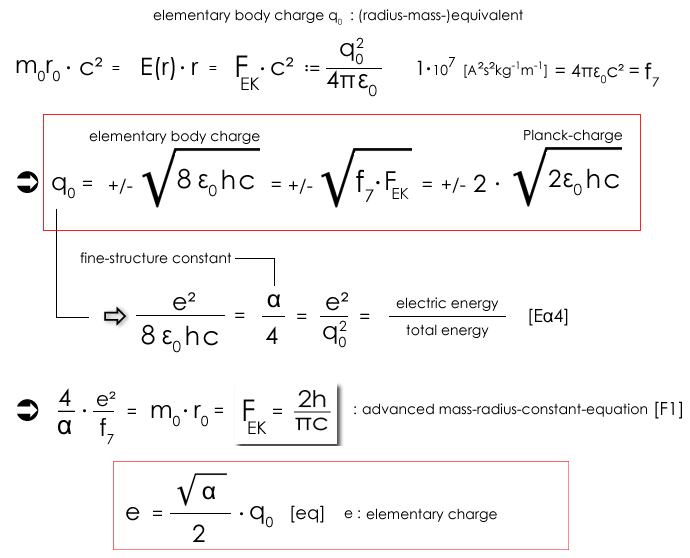f7 was "introduced" to show that the [elementary body] charge q0 is ("only") a scaled mass-radius function.

Side note

Particle physicists generally use the phenomenologically incorrect term decay even though they mean transformation. Decay would mean that the decay products were (all) components of the original particle. But that is not the case, at least not within the theoretical implications and postulates of the Standard Model of particle physics (SM).

 For convenience, "powers of ten" are written as follows: 1.23 · 10-3 = 1.23e-3. This has the advantage that the interested reader can take over the corresponding numerical values in the "computer" for "control" (copy & paste). The uncertainties of the (CODATA) (mass) values and the natural constant values are not explicitly named. Example: Mass of the electron: 9,10938356e-31 kg 9,10938356 (11) e-31, means a standard deviation of ± 0,00000011e-31 kg. It is calculated here with the value 9,10938356e-31 kg.

Charge-dependent matter formations

Basics

The extended charge principle leads beyond elementary-body theory-based hydrogen atom-forming to additional proton-electron interactions. From the generalized, clear phenomenological process stringently follow the neutron (e-q0 interaction) and pions (q0-q0 interaction) as energetically possible (time-instable) "particles". Without concretizing this here, the charged pions “decay” (convert) into muon and anti-muon and then into electron and positron. Overall, "diverse elementary particles" can be formed in the context of the extended charge concept in "formal analogy". Noteworthy is the fact that this formalism provides simple, without free parameters solutions that are in good agreement with the (energy and mass) values of the "formed particles".

On the basis of the reduced mass of the electron it can be easily shown how a model view "works wrongly". Considering celestial mechanics, a "small" centroid shift results from the proton to the electron, since the mass of the proton is finite. From the point of view of two equal charges, this assumption is unfounded, since masses in the standard view of physics only have an effect via the (misunderstood) gravitation, which is smaller by almost forty powers of ten to the electrical force. Overall, in the world view of the prevailing physics, a mass can not interact with a charge, because there exists simply no such phenomenology. It is astonishing how this fact was ignored mass psychologically over generations and is still ignored.

... there is no mass-center-shift "between two equal I charges I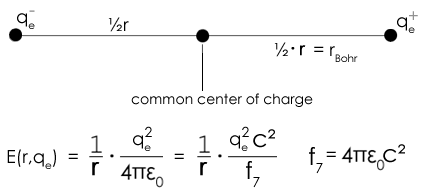BUT : The model view that the interaction between "charges" that are at a distance r from each other does not occur in matter-forming elementary-body theory. In elementary-body theory, the "charges" overlap with/in a common origin.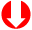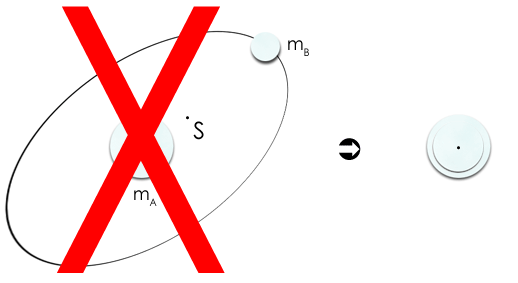Reduced mass alternative in a mass-space-coupled model

Let's start with the superposition of two elementary bodies A and B, with the masses mA and mB and the mass-coupled radii rA and rB. By mass-radius-constant equation [F1] there is no "room" for interpretations ► The result applies to all charge carrier constellations (... A-B, proton-electron, proton-muon, ...)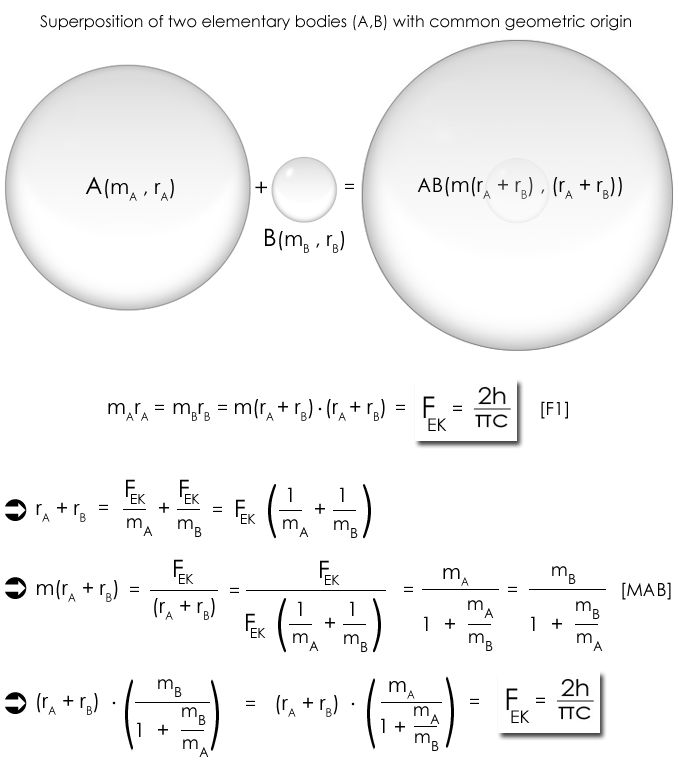Here one can clearly see that the alleged center-of-gravity correction of the "celestial-mechanical model has nothing to do with  (for example proton and electron) with focus on two interacting charges (at a distance r), since electron and proton, as equal charges, can not undergo a shift, either phenomenologically or computationally.

NOTICE! Equating an electrical centripetal force with a (only) mass-dependent centrifugal force is phenomenologically unfounded in the context of physics and is reminiscent of the epizykel theory. The expression for the resulting mass m(rA + rB) in equation [MAB] is mathematically identical to the celestial-mechanical centroid correction of two macroscopic masses (reduced mass) which computationally interact elastically as point masses, but the phenomenology for the equation [MAB] is a completely different one.

Furthermore, the calculation of ground state energies is neither quantum mechanically nor quantum electrodynamically possible. Since a significant amount is determined by the ratio of the interacting masses. There is neither QM nor QED based the possibility of introducing the reduced mass mred = mA / (1 + mA / mB) quantum-field-phenomenologically. The reduced mass - whether one wants it to be true or not - is historically derived from "Newtonian celestial mechanics" within the framework of standard physics. This means in plain language that in terms of atomic interactions, these are neither QM nor QED justified. QM and QED are "epicyclic".

 Mass - inherent radius (of the electron) re The SM-based postulated theoretical implications strongly influence the experimental interpretations of the SM protagonists ... The resulting complex expectation construct leads to a series of fatal misunderstandings. By way of example, the "typical" energy-dependent electron radii, less than 10-19 [m], in connection with particle accelerators are not "false" (measured), but the inferences concerning resting electrons resulting from elementary particle physics are false. The elementary-body theory (on the other hand) consistently describes both the behavior of the "conventional" scattering energies of the scattering partners (of the electron) and of high-energy particles in accelerators.   [Practical note: If you can't "follow" respectively won't accept logic based facts, consider the "following"...  Since almost all successfully SM-indoctrinated people get a crisis by „hearing“ the term electron radius and "instantaneously" think of the unpopular classical electron radius, they should “replace” the electron mass inherent radius (re) through the "problem-free" Compton wavelength of the electron (λe) and postpone their "communication problem". Don't dramatize the model view : λe = ˝ · π  · re]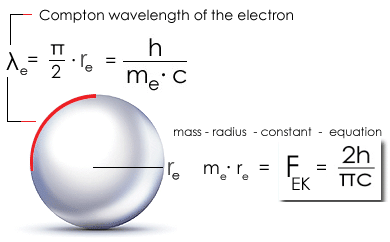First of all, the differential scattering cross section dσ/dΩ always comprise the form : dσ/dΩ = (e˛/me4πε0c˛)˛ · fW for electrons in "conventional" scattering experiments.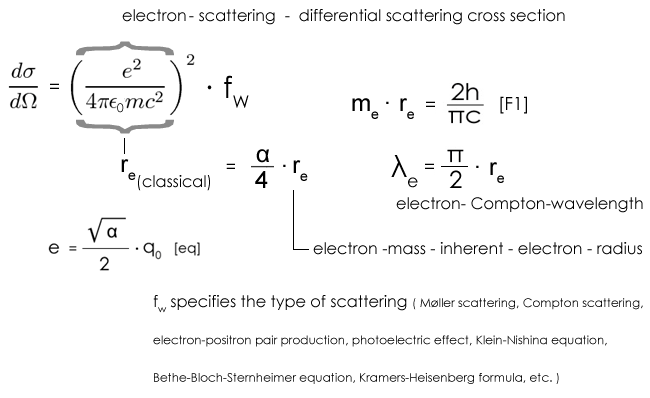fW indicats the specific type of scattering. The „communication problem” of the prevailing physics with respect to the (unpopular) classical electron radius re(classical) is resolved by analyzing the recurrent mass-radius-coupled term (e˛/me4πε0c˛) energetically. Finally, the term (e˛/me4πε0c˛) consists of the ratio: electrical energy to total energy (α/4) and the electron mass inherent electron radius re [ = (dσ/dΩ) / ((α/4) · fW)], multiplied by π/2 corresponds to the Compton wavelength of the electron (λe = ˝ · π  · re).  Furthermore, the object radius changes with the velocity radially symmetric and not just one-dimensional in the direction of movement. Here, too, elementary-body theory provides a phenomenologically-based, consistent, realobject-oriented model.  One can not mention it often enough: Apart from "SM-based interpretations" of particle accelerator physics, the electron mass inherent electron radius re respectively the classical electron radius re(classical) = ((α/4) · re), is used in all (!) equations for the calculation of the scattering cross sections for elastic and inelastic scattering of electrons (keywords: Mřller scattering, Compton scattering, electron-positron pair production, photoelectric effect, Klein-Nishina equation, Bethe-Bloch-Sternheimer equation, Kramers-Heisenberg formula, etc .). re has a phenomenologically based connection with the Compton wavelength (of the electron). Here, so to speak, "avenges" the lack of a consistent, phenomenologically motivated (standard physics) concepts of electric charge. See the various mathematical approaches of classical electrodynamics, quantum electrodynamics (QED), quantum chromodynamics (QCD) and in particular the electroweak theory. In the context of elementary-body theory, the electric elementary charge (e) and the associated electrical energy E(e) expresses a mass-radius-coupled energetic ratio. For this »understanding problem« exists the biggest emotional outbursts and a methodical plausibility refusal from standard physicists and their followers (... more details see the chapter electron radius).

Charge-dependent matter formation possibilities

The charge-dependent matter formation generally describes the A-B interaction possibilities. A and B are elementary bodies with the masses mA and mB and the reciprocal proportional radii rA and rB. The following applies: mA · rA = mB · rB = FEK = 2h / πc  [F1]. The phenomenologically founded formalism leads to the equations: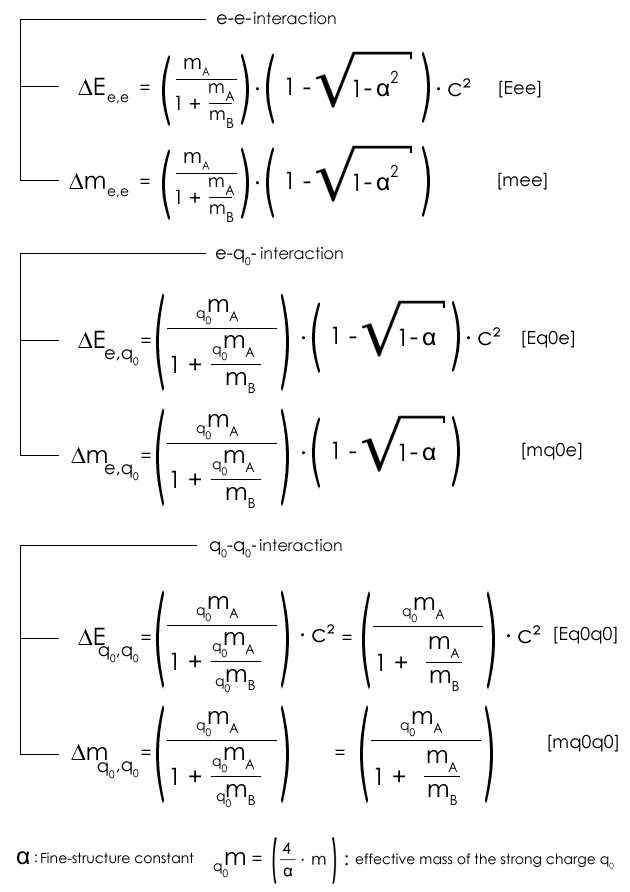In the above (matter-forming) α-function-equations, only the masses mA and mB of the interacting elementary bodies occur as variables. The charge as such, or more precisely the charge size, is implicitly determined by the functional relationship of the Sommerfeld fine structure constant α. (Details and derivations see »Ladungsabhängige Materiebildungen«)

e-e interaction

The term e-e interaction means that two elementary charge carriers interact. The most prominent example of this type of interaction is the proton-electron-based hydrogen atom.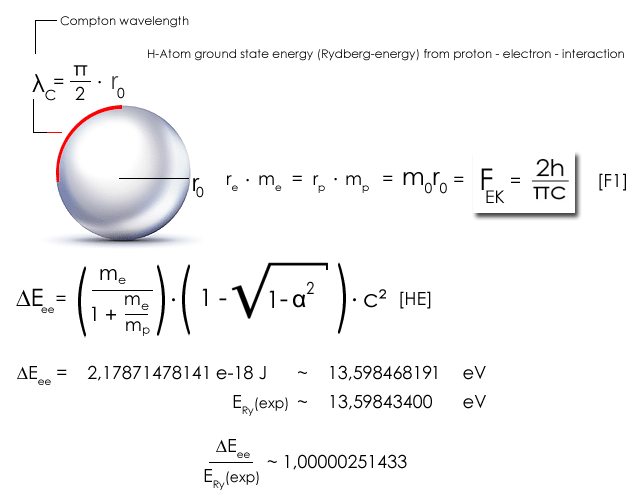mA  = me = 9,10938356e-31  kg : electron-mass        mB  = mp = 1,672621898e-27  kg  : proton-mass

c =  2,99792458e+08 m/s  α = 0,0072973525664

e-q0 interaction

elementary body carrier A(q0) interacts with elementary carrier B(e). The most prominent example of this type of interaction is the proton-electron-based neutron.

The neutron mass mn arises from a matter-forming charge interaction of the electron and proton and can be understood and calculated by the interaction of the elementary body charge q0 for the electron and the elementary electric charge e for the proton.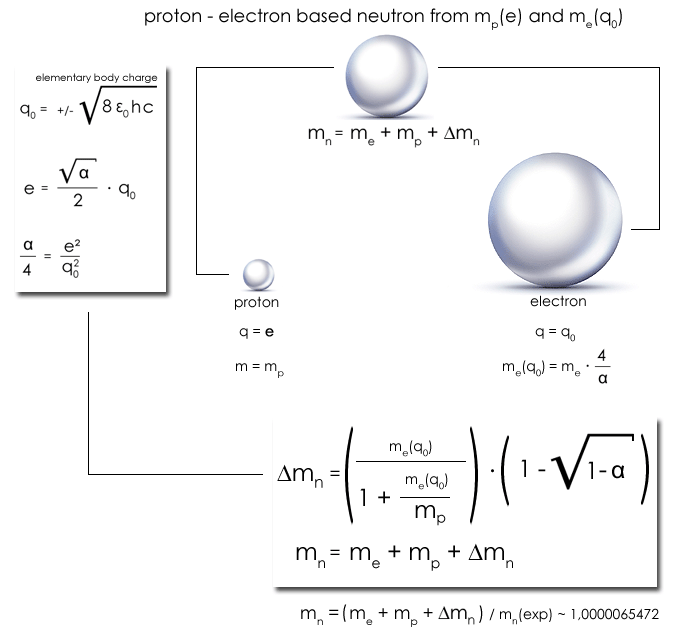me = 9,10938356e-31  kg        me(q0) =   (4/α) · me =  4,99325391071e-28 kg        c = 2,99792458e+08 m/s

mp 1,672621898e-27 kg

∆m = 1,405600680072e-30 kg    ∆Eee =    1,263290890450e-13 J      ~    0,78848416 MeV

Taking into account the phenomenologically-based, approximation-free approach, in formal-analytic form of the equation: mn = mp + me + Δm [mq0e], the "theoretical" result of elementary particle theory based neutron mass calculation (according to charge-dependent proton-electron interaction) is “sensational”. In addition you'll find below a calculation of the magnetic moment of the neutron  (see SUMMARY of FORMULAS).

q0-q0 interaction

Charge carriers A and B interact via the elementary body charge q0. The most prominent example of this type of interaction is the proton-electron-based charged pion.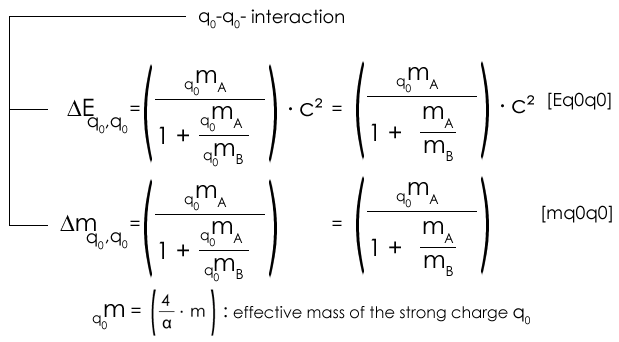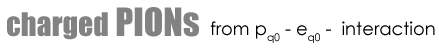mA  = me = 9,10938356e-31  kg : electron-mass

q0mA =   (4/α) · me =  4,99325391071e-28 kg

mB  = mp = 1,672621898e-27  kg  : proton-mass

q0mB =   (4/α) · mp =  9,16837651891e-25 kg

c = 2,99792458e+08 m/s      α = 0,0072973525664[mq0q0]

∆m = 4,99053598e-28  kg     (∆m/2) / mπ(exp)  ~  1,00289525

... ∆m means the mass of two charged pions (matter creation)

The extent to which experimental particle physics can accurately determine resting pion masses is highly doubted. The neutral pion is a "pion" due to the different mass of the charged pions only in the SM requirement. The abstraction, which is "equal" to particles with different masses according to postulated QM superpositions (keyword: quarkonia), is one of the many arbitrariness hypotheses within SM (see SM-quark mass uncertainty in the percent error range) and "outside" of mathematical formalism of the SM unfounded.

For mass-like interaction partners (for example, proton-antiproton or electron-positron) the general α-function equations simplify - for example the  q0-q0 interaction - to the equations ([Eq0q0] and [mq0q0]):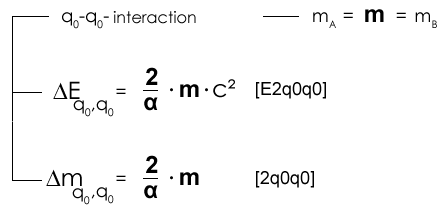"Surprising" is the "circumstance" that in the context of "charge-dependent matter formation the strong proton-antiproton interaction follows a matter-formation energy of ~ 257 GeV depending on the (anti) proton mass and the Sommerfeld fine-structure constant α which, according to charge conservation, produces as a variation possibility two mass-radius-coupled "small mass heaps" (Masse-Häufchen) which map uncharged and charged Higgs-boson masses. The final equation [2q0q0] is unbeatable in terms of simplicity.

α  = 0,0072973525664m := mp = 1,672621898e-27  kg  : (anti-)proton-mass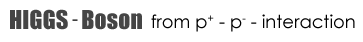∆E( p+, p- )  =  257,15410429801 GeV

∆m( p+, p- )   =   4,584188259456e-25   [kg]  [2q0q0]

(∆m( p+, p- ) / 2) = 128,57705215 GeV/c˛

mH(0)  ~ 2,228e-25 kg   ~  125 GeV/c˛

(∆m( p+, p- ) / 2) / mH(0)  1,02861642

This means that with an "error" ~ 2.9%, based on the Higgs boson mass "detected" at the LHC (mH(0) ~ 125 GeV / c˛), elementary particle theory predicts an event which exists in the standard model of Particle physics (SM) only as a theoretically predetermined methodical circular conclusion.

by the way ...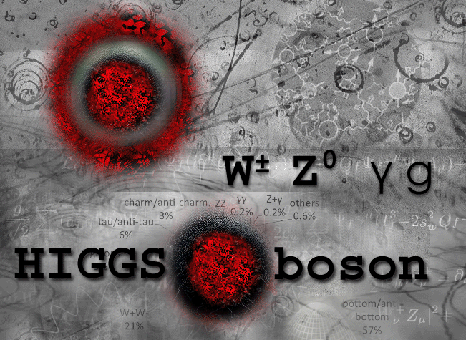There are aspects of the Higgs-Boson-mass predictions which are barely known.

David and Sidney Kahana's predictions about the Higgs-Boson-mass and the Top-Quark-mass (1993!!!) in a “parameter free fashion” are very precise. Source: https://arxiv.org/pdf/hep-ph/9312316.pdf

According to the standard model (SM) predictions are not possible. How do you explain the obvious discrepancy?

Peter Higgs knew about their work … he said, “You’re from Brookhaven, right. Make sure to tell Sid Kahana that he was right about the top quark 175 GeV and the Higgs boson 125 GeV” [Kahana and Kahana 1993].”… Source:https://arxiv.org/pdf/1608.06934.pdf

One would assume that highly accurate calculations about the Top-quark-mass and the Higgs-mass are remarkable. Why didn’t the “Kahanas” get the “proper” attention? Why is there no adequate mention about these theoretical achievements?

I strongly believe that Sidney and David Kahana’s predictions need to be published and discussed again. With reference to Nambu Y and Jona-Lasinio G 1961 Phys. Rev. 122 (1) 345-358 (https://journals.aps.org/pr/pdf/10.1103/PhysRev.122.345)  it seems that the entire SM-project had already been completed (…“methodical circular conclusions") at the beginning of the 1960s.

For further reading see https://arxiv.org/pdf/1112.2794.pdf ... "predictions by the authors D. E. Kahana and S. H. Kahana , mH = 125 GeV/c˛ uses dynamical symmetry breaking with the Higgs being a deeply bound state of two top quarks. At the same time (1993) this model predicted two years prior to the discovery to the top its mass to be mt = 175 GeV/c˛..."

Notice! There is just one outstanding »prediction paper« (1993 https://arxiv.org/pdf/hep-ph/9312316.pdf) which leads to the Higgs-Boson-mass and the Top-Quark-mass with the same theoretical approach prior to the experimental confirmation in 1995 (Top) and 2012 (Higgs).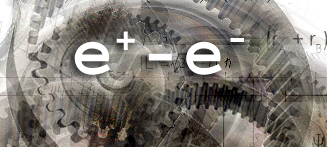The uncharged pion ... a pion matter possibility from q0 - q0 interactionmA = mB = me± = 9,10938356e-31  kg : electron-mass = positron-mass

q0mA =   (4/α) · me =  4,99325391071e-28 kg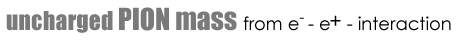∆E( e+, e- )    =      140,05050232093 MeV    [E2q0q0]

∆m( e+, e- )         =   2,496626955355e-28   kg     [2q0q0]

2,4061764315e-28 kg      mπ0  SM - theory laden

∆m( e+, e- ) / mπ0  ~ 1,037591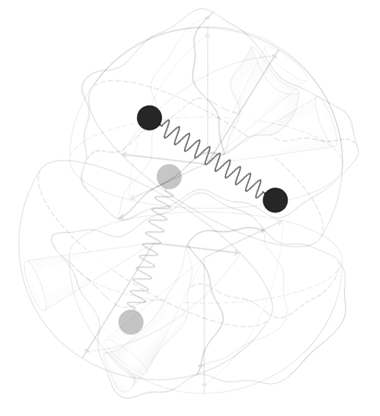Wishful thinking and reality

I find it quite amusing and right to the point how Claes Johnson, a Professor of Applied Mathematics, classifies ...

Claes Johnson about QM and SRT

Concerning the crisis of modern physics it is commonly accepted that one reason is that the two basic building blocks, relativity theory and quantum mechanics, are contradictory/incompatile. But two theories which are physical cannot be contradictory, because physics which exists cannot be contradictory. But unphysical theories may well be contradictory, as ghosts can have contradictory qualities.

The Special Theory of Relativity of Einstein is unphysical because the Lorentz transformation is not a transformation between physical coordinates, as strongly underlined by its inventor Lorentz, but misunderstood by the patent clerk Einstein believing that the transformed time is real and thus that time is relative. Quantum Mechanics is unphysical because its interpretation is statistical which makes it non-physical, because physics is not an insurance company. Here Einstein was right understanding that God does not play dice.

Professor of Applied Mathematics, Royal Institute of Technology (KTH) Stockholm , Sweden

Claes Johnson Blog

______________________________________________Albert Einstein

It could be helpful to remember what Albert Einstein wrote on quantum mechanics:  "The ψ function is to be understood as a description not of a single system but of a system community [Systemgemeinschaft]. Expressed in raw terms, this is the result: In the statistical interpretation, there is no complete description of the individual system. Cautiously one can say this: The attempt to understand the quantum theoretical description of the individual systems leads to unnatural theoretical interpretations, which immediately become unnecessary if one accepts the view that the description refers to the system as a whole and not to the individual system. The whole approach to avoid 'physical-real' becomes superfluous. [Es wird dann der ganze Eiertanz zur Vermeidung des ‘Physikalisch-Realen’ überflüssig.]  However, there is a simple physiological reason why this obvious interpretation is avoided. If statistical quantum theory does not pretend to describe completely the individual system (and its temporal sequence), then it seems inevitable to look elsewhere for a complete description of the individual system. It would be clear from the start that the elements of such a description within the conceptual scheme of the statistical quantum theory would not be included. With this, one would admit that in principle this scheme can not serve as the basis of theoretical physics.”

 A. Einstein, Out of my later years. Phil Lib. New York 1950 Seite 498

According to the Copenhagen interpretation of 1927, the probability character of quantum theoretical predictions is not an expression of the imperfection of the theory, but of the essentially indeterministic (unpredictable) character of quantum physical natural processes. Furthermore, the "objects of formalism" "replace" reality without possessing a reality of its own.

The Copenhagen interpretation is characterized by the „comfort” it provides to its "believers”. The wave-particle dualism allows a "transition" to the "wave" with an e-function with complex exponent, which according to Fourier theorem in turn allows "ALL" piecewise monotonous, so also any experimental result to represent formally mathematical. The statistical interpretation refrains from the effort to explore the physical process, because in principle only probability statements in quantum processes are possible

In the time after the Second World War, the Copenhagen interpretation had prevailed, in textbooks was now only the Heisenberg-Bohr quantum theory without critical comments to find.

___________________________________________The Quark Parton Model (QPM), developed by Richard Feynman in the 1960s, describes nucleons as the composition of basic point-like components that Feynman partons called. These components were then identified with the quarks, postulated by Gell-Mann and Zweig at the same time a few years earlier. According to the Quark-Parton Model, a deep inelastic scattering event (DIS deep inelastic scattering) is to be understood as an incoherent superposition of elastic lepton-particle scattering processes.

A cascade of interaction conjectures, approximations, corrections, and additional theoretical objects subsequently "refined" the theoretical nucleon model.

A fundamental (epistemological) problem is immediately recognizable. All experimental setups, implementations, and interpretations of deep elastic scattering are extremely theory based.

Fundamental contradictions exist at the theoretical basis of the Standard Model of particle physics, which, despite better knowledge, are not corrected. An example:

The nonexistent spin of quarks and gluons

A landmark, far-reaching wrong decision was made in 1988.

The first assumption was, due to the theoretical specifications of the mid-1960s that in the image of the SM the postulated proton spin is composed to 100% of the spin components of the quarks. This assumption was not confirmed in 1988 in the EMC experiments. On the contrary, much smaller, even zero-compatible components were measured (ΔΣ = 0.12 ± 0.17 European Muon Collaboration). Also the next assumption that (postulated) gluons contribute to the proton spin did not yield the desired result. In the third, current version of the theory, quarks, gluons (...virtual Quark-anti-Quark pairs if one wishes too) and ... their dynamical-relativistic orbital angular momentum generate the proton spin.

On closer inspection, the second readjustment has the „putative advantage” that the result in the context of lattice gauge theory and constructs, such as "pion clouds", algorithmically "calculated", can’t be falsified. But this purely theoretical based construction obviously does not justify the classification of quarks as fermions. No matter how the asymmetrical ensemble of unobservable postulated theoretical objects and interactions is advertised and will be advertised in the future, the quarks themselves were never "measured" as spin-˝ particles.

Summary in simple words: It is possible to create a theory-laden ensemble of Quarks and “other” theory objects and their postulated interactions, but the Quark itself - as an entity - has still no intrinsic spin -˝ in this composition. That means that Quarks aren’t fermions, no matter what the actual theoretical approach would be! This is a basic, pure analytical and logical statement.

Generally speaking: If one postulates a theoretical entity with an intrinsic value but one discovers that one needs to add theoretical objects and postulated interactions to get the desired intrinsic value, one has to admit that ones entity has no physical characteristic as such.

Further more:

In sum, the quark masses postulated according to the SM do not yield the nucleon masses by far. Gluons are massless.

Postulated Up-Quark mass: 2.3 ± 0.7 ± 0.5 MeV / c˛ up (u)

Postulated down-quark mass: 4.8 ± 0.5 ± 0.3 MeV / c˛ down (d)

938,272 0813 (58) MeV / c˛ Proton mass duu ~ 0,8 - 1,2% (!!!) Quark mass fraction

939,565 4133 (58) MeV / c˛ neutron mass ddu ~ 1,1 - 1,4% (!!!) Quark mass fraction

Thus, also heavy ions composed of protons and neutrons (such as lead or gold nuclei) can not be represented by quarks and gluons. This means that according to the principle of mass-energy equivalence, nucleons and, ultimately, heavy ions consist almost entirely of phenomenologically indeterminate binding energy. Even more complicated is the fact that the ions are accelerated to almost the speed of light before they collide. This means that there is also a considerable amount of external energy added to the binding energy. Neither the theory of relativity neither the SM does tell us how these phenomenologically can be divided into translational energy and "mass equivalence."

Protagonists of the SM are so convinced of their belief that they have obviously lost sight of the essential. Why should a postulated complex, multi-object-asymmetric, charge-fragmented, dynamic substructure create a spin value ˝ and an elementary charge of exactly 1·e over dynamic states in the temporal or statistical mean? The comparison with the SM point-postulated, "leptonic" electron, with spin value ˝ and elementary charge 1·e, which are "created" without "dynamic effort" and structure, identifies the quarks-gluon thesis as a fairy tale.The "fragmentation of matter" as an »end in itself« of mathematical theories and the inevitable increase of irrelevant knowledge, especially in the form of virtual particles, has become established standard thinking. Instead of simplification, the concepts of formal postulations and "refining theories" obviously do not end in the growth of knowledge but in scientific arbitrariness. Mathematical-based fundamental physics urgently requires a natural-philosophical oriented regulation.

Unfortunately there is no complete English translation for the "Elementarkörpertheorie" yet available. You'll find more detailed information if you select certain main issues from the website menu (auf Deutsch). "Feel free" to use a common webbrowser translation tool. You'll discover useful information, insights and surprising equations to deduce and calculate physical values based on mass-radius-relations such as... Sommerfeld Fine-structure constant, neutron mass, mass(es) of charged pions, mass and radius of the universe, Planck units, cosmic microwave background temperature, ...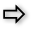[ Email-contact: Dirk Freyling : dfreyling@fastmail.net ]

... "for now" you'll find "here" (more) important results and a short discription of how to gain those below in the summary of formulas.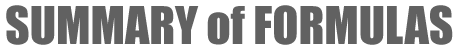Anatomy of anomalous magnetic moments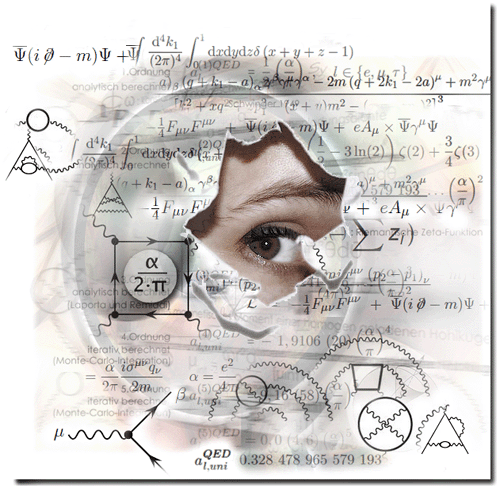The entire analysis of (anomalous) magnetic moments is qualitatively and quantitatively very extensive. Here - more or less - only the results are presented in English. Detailed derivations and justifications can only be found in the German version, see Anatomie anomaler magnetischer Momente.

Noteworthy is the fact that the experimental results, if considered without the theory-laden expectations of "leptonic" structure-less "or quark-based substructures, have an easily identifiable commonality: the additional (supposedly anomalous) magnetic moment contribution to the semiclassical for the proton and electron is ~ 1 · 10-26 Joule/Tesla.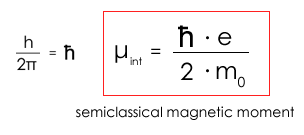[μB´(th)]

h = 6,626070040e-34  Js     e = 1,6021766208e-19  As      me = 9,10938356e-31 kg       mp = 1,672621898e-27 kg

∆μB (p)   =  1,41061e-26 J/T  [μexp]     -    5,0507837e-27  J/T  [μBp(th)]       ~   0,90553e-26    J/T

∆μB(e)    =  9,28477e-24 J/T  [μexp]     -    9,27401e-24   J/T     [μBe(th)]      ~    1,075463e-26  J/T

In other words, if one embodies the magnetic field in an "energetic analogy", the metrologically recorded magnetic moment of the proton and the electron result from the energetic superposition with the magnetic field. The magnetic field itself as "energy generator" interacts with electron and proton and provides a measurement-inherent, coupled contribution of the order of 1e-26 J/T to the measured magnetic moment of the object to be "examined". This means that the entire mathematical QFT-magic around supposedly anomalous (intrinsic) magnetic moments and their "leptonic" QED corrections are theory-induced, or simply formulated - in the truest sense of the word - irrelevant. Furthermore, it follows that the experimentally determined magnetic moment of the proton is now plausible without a substructure.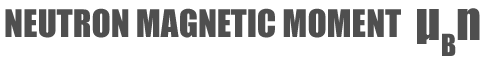Are there, besides the obviously plausible argument, that one can not simply ignore the energy contribution of the magnetic field (as usual in QM, QED and QCD), any further indications for an additive contribution to the magnetic moment of the tested particles? It is the magnetic moment of the electrically uncharged neutron, which does not exist in the semiclassical view and gets it magnetic moment of the postulated neutron substructure. But is the magnetic moment of the neutron really a proof of a substructure? Or is this assumption just a theory-laden interpretation of the standard model?

A look at the "naked" numbers confirms the thesis that the magnetic moment of the electrically neutral (proton-elcetron-based) neutron results exclusively from the magnetic field contribution that the neutron induces in the (applied) magnetic field:

∆μBn    =                        μBn(exp)               -    μBn(th)       =     9,6623650e-27 J/Tesla      μBn(exp)  =  ∆μBn

9,6623650e-27 J/Tesla     -   0   J/Tesla

Consistent assumption: The measured value μBn(exp) ~ 9,66237e-27 J/T for the (electron-proton-based) neutron magnetic moment is "nothing more" than the measurement-inherent contribution of the magnetic field generated by elementary body-based matter-forming (charge-internal) interaction of electron and proton, "induced" in the magnetic field. Which also means that only the neutron in a magnetic field has a magnetic moment!

"Proof": If the assumption is right, then the magnetic moment of the electron-proton-based neutron (μBn(exp) = ΔμBn) must be calculated from the measurement-inherent magnetic field contributions of electron and proton (ΔμBe and ΔμBp). A "simple" way to connect the three quantities ΔμBn, ΔμBe and ΔμBp without explicit knowledge of the magnetic field embodiment is: (ΔμBn) ˛ equate to ΔμBe · ΔμBp. Here it must be taken into account that the neutron is composed of the q0-electron and e-proton charge interaction."just remember"...

The neutron mass mn arises from a matter-forming charge interaction of the electron and proton and can be understood and calculated by the interaction of the elementary body charge q0 for the electron and the elementary electric charge e for the proton.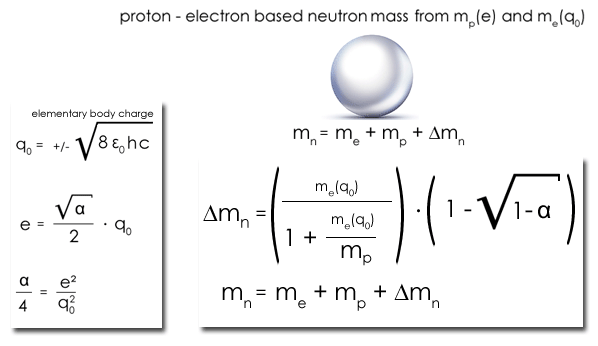me = 9,10938356e-31  kg        me(q0) =   (4/α) · me =  4,99325391071e-28 kg        c = 2,99792458e+08 m/s

mp 1,672621898e-27 kg

∆m = 1,405600680072e-30 kg    ∆Eee =    1,263290890450e-13 J      ~    0,78848416 MeV

Taking into account the phenomenologically-based, approximation-free approach, in formal-analytic form of the equation: mn = mp + me + Δm [mq0e], the "theoretical" result of elementary particle theory based neutron mass calculation (according to charge-dependent proton-electron interaction) is “sensational”.This can be expressed by the factor 1 + (e/q0) = (1 + (√α/2)). The resulting - consistently phenomenologically justified - result [equation μn] is remarkable...............................................................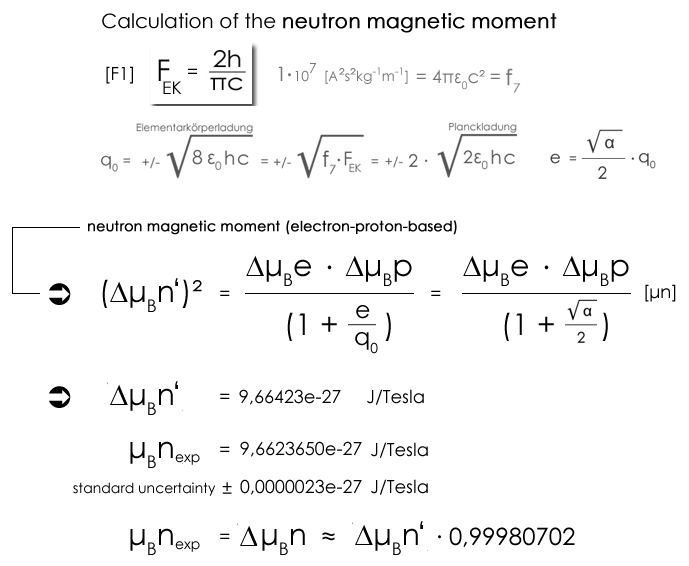Equation [μn] can be "refined" phenomenologically, by including an explicit mass dependence of the neutron in the calculation, which expresses the effective charge-dependent mass reduction (inherently coupled to a charge-dependent proportional charge-radius magnification) in relation to the total neutron mass. Similar to the hydrogen atom, the object radius increases in dependence of the charge, only that in the case of the neutron the proton interacts as an elementary body carrier e (e-p) with the electron as an elementary body carrier q0 (q0-e). Furthermore, the neutron energy as such is "conserved" overall, whereas the H-atom emits half of the total energy as (α/4) -scaled binding energy. The result for the neutron is the factor 2 for the effective charge mass in comparison to the total neutron mass.

phenomenologically based "refined" calculation of the neutron (anomalous) magnetic moment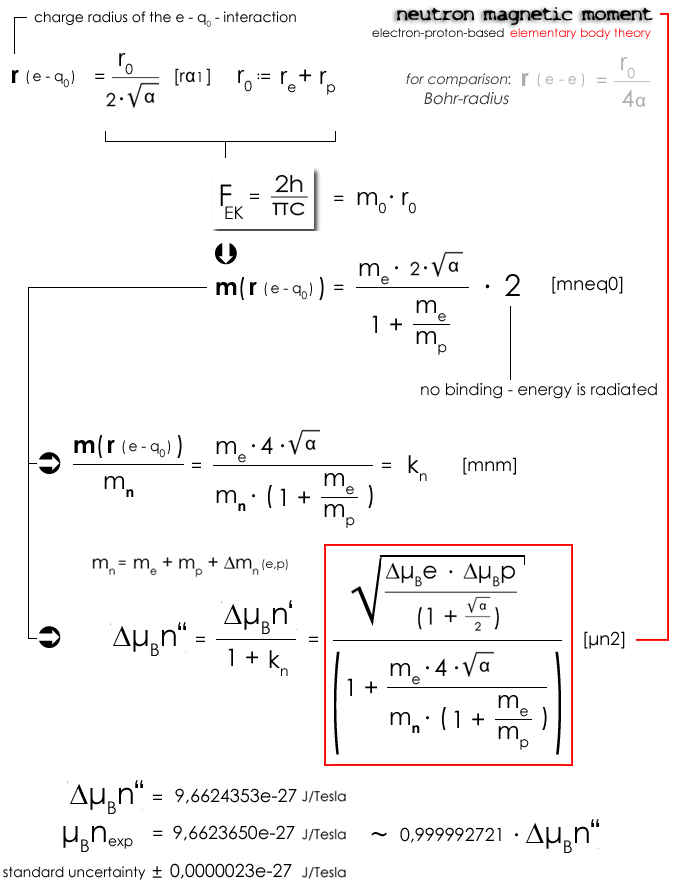compare with CODATA  neutron magnetic moment

Conclusion

The consistently phenomenologically-based, formalized prediction of the magnetic moment of the neutron (equations [μn] and [μn2]) based on charge-interaction magnetic contributions of electron and proton (ΔμBe and ΔμBp), identifies the neutron as electron-proton-based. The magnetic moment of the neutron is a pure "magnetic field embodiment", meaning: The "magnetic field free" neutron has - compared to proton and electron - no intrinsic magnetic moment, μBn(exp) consists solely of the magnetic field measurement inherent contribution ΔμBn.

∆μBn    =                        μBn(exp)               -    μBn(th)       =     9,6623650e-27 J/Tesla      μBn(exp)  =  ∆μBn

9,6623650e-27 J/Tesla     -   0   J/Tesla

Anomalous magnetic moments of proton and electron

”Quantum electrodynamics is not a foundational theory of natural philosophy because it obtains the right result by arbitrary means: dimensional regularization, which changes e, and renormalization, which artificially removes infinities of the path integral method. Quantum electrodynamics is Lorentz covariant only (it is a theory of special relativity). Quantum electrodynamics uses the sum over histories description of the wavefunction. This is an acausal description in which the electron can do anything it likes, go backwards or forwards in time for example. This acausality or unknowability Anomalous Magnetic Moment of the Electron is contradicted fundamentally and diametrically in QED by use of the Huygens Principle, which expresses causality or knowability - the wavefunction is built up by superposition in causal historical sequence - an event is always preceded by a cause, and nothing goes backwards in time. For these and other reasons QED was rejected by Einstein, Schrödinger, de Broglie, Dirac and many others from its inception in the late forties.”…

To get an impression of the fundamental theory problem of "radiation corrections" in a historical context, we recommend the following contribution of Mario Bacelar Valente: The renormalization of charge and temporality in quantum electrodynamics

Using concrete examples, Valente shows how result-oriented, partly arbitrary "mathematical extensions and transformations" are included in the calculations and how "here and there" terms are declared to be unphysical and their divergences are not taken into account. This is highly problematic, since no binding axiomatic rules apply. It also becomes clear that there are no physical interpretations that fill the mathematical procedures with phenomenological content."

 Magnetic moments - the experimental site ... The Penning trap is a device for the storage of charged particles using a homogeneous axial magnetic field and an inhomogeneous quadrupole electric field to measure magnetic moment. This type of "ion cage", in which the oscillatory motion of individual particles can be detected and controlled with great accuracy, has become established as a measuring instrument for high-precision measurements thanks to the work of Hans Georg Dehmelt (Nobel Prize 1989). Idea: By superimposing an electric 3D multipole field with a magnetic dipole field, it is possible to "fix" an ion. In this case, the magnetic field applied in the z-direction prevents the ion from breaking out in the radial direction, while the electric field ensures the axial confinement. The first experiments of this kind go back to Frans Michel Penning in the 1930s. See exemplary :

Regarding the magnetic moment of the electron today's desired predictability equates to the distance Earth-Moon with proverbial hair-width accuracy. Metrologically, the question arises as to whether the information has been lost, that the propagated measurable "mirror current" or "spin-flip" of individual electrons and protons, for example in the double Penning trap, are influenced by the measuring apparatus as a "quantum mechanical observer".

The basic "modern" misunderstanding of interpreting a (quantum mechanical) experiment with "idealization" or “reduction” means that the experimental setup - which "provides" additive energy in the form of electric or magnetic fields - does not act as an (energetic) interaction partner. But supposedly intrinsic “values” ​​of objects, such as the fine structure of the spectral lines, or magnetic moments, are partly “created” by the "application of external fields". This logically comprehensible unavoidable "observation effect" is categorically denied respectively ignored by the protagonists of standard-model physics.

Although the accuracy requirements and corrective measures are understandable, it remains the suspicion of complex idealizations that produce the results that go far beyond the capabilities of macroscopic experimental setups. In relation to a single electron or proton, it is easy to comprehend that "everything" is - so to speak - macroscopically. It would be nice if researchers of the Penning trap experiments are less influenced by theory-laden thinking and much more analytic.

Detached from historical quantum mechanical fantasies (the Copenhagen interpretation) and resulting inherent observer-observation object-interactions that influence the measurement result, as well as subsequently adventurous-precise measurement constellations, the overarching natural-philosophical basic problem is quickly named. There is simply no decoupling between theoretical conception and experimental realization and no consensus on the anatomy of the measurer from the point of view of prevailing physics. Means: The measurements are heavily theorie-laden and considering that the propagated theory objects belonging to the measurement are a wild mixture of classical and quantum field theoretical terms and sizes. The plausible and logically comprehensible statement that both the (applied) electric field and the magnetic field, even contribute to the supposedly intrinsic magnetic moments of the charge carriers to be examined, is suppressed by the QED-theorists and experimenters.

Based on the experimental values for the magnetic moments of electron, proton and neutron, it is concluded that the magnetic field itself provides an additive, measurement-inherent contribution to the supposedly intrinsic values.

First conspicuties

It is noticeable that the experimental values of the magnetic moments (μBe(exp)/μBp(exp) ~ 658,2107) are significantly different, but the absolute difference values ΔμBe and ΔBp to the theoretical values μBe(th) and μBp(th) are similar.

This means: If one subtracts from the experimental value of the magnetic moment of the proton μBp(exp) the (semiclassical) "theoretical" expectation μBp(th) (equation [μintm]) and compare this difference with the experimental value of the magnetic moment of the electron μBe (exp) minus the "theoretical" value of the magnetic moment of the electron μBe(th), it is found that these are "order of magnitude similar" (ΔμBe / ΔμBp ~ 1.19 / 1).

... additive  [J/Tesla] - magnetic field contributions to the proton, neutron und electron magnetic moments  ...∆μBp         ~          ∆μBn                ~      ∆μBe                [ ! ]

9,055284175e-27    ~      9,6623650e-27              ~ 1,075462794596e-26

1                :           1,06704161                 :        1,18766322

numerical values

electron  m0(e) = me =  9,10938356e-31 kg      r0(e) = re = 2h/(πcme) = 1,5446370702e-12  m      λC(e)  = λe = (π/2)  · re

9,27400999205404e-24 J/Tesla    μBe(th)  (semiclassical) theoretical value of the electron magnetic moment

9,284764620e-24  J/Tesla   μBe(exp)  measurement of the electron magnetic moment

(-) 2,00231930436182 [CODATA2014] ge  electron g factor

μBe(exp) = ( 1 + 0,00115965218091) · μBe(th)    ►   fe = 0,00115965218091

1,075462794596e-26 J/Tesla  : difference value  μBe = μBe(exp) - μBe(th)

__________________________________________________________________________

proton  m0(p) = mp =  1,672621898e-27 kg      r0(p) = rp = = 2h/(πcmp) = 8,412356403e-16  m      λC(p)  = λp = (π/2)  · rp

5,0507836982111e-27 J/Tesla    μBp(th)  (semiclassical) theoretical value of the proton magnetic moment

1,4106067873e-26    J/Tesla   μBp(exp) Meßwert, magnetische Moment des Protons

5,585694702 [CODATA2014] gp  Proton g Faktor

μBp(exp) = ( 1 + 1,7928473512) ·μBp(th)    ►   fp = 1,7928473512

9,0552841747889e-27  J/Tesla  : Differenzwert  μBp = μBp(exp) - μBp(th)

__________________________________________________________________________

electron-proton-ratios

1836,15267376007   =  mp/me  = μBe(th) /  μBp(th)

2,78961237051261160  =  ( mp/me )  /  ( μBe(exp) / μBp(exp) )  = ( μBe(th) / μBp(th) )  /  ( μBe(exp) / μBp(exp) )

658,21068660613  =  μBe(exp) / μBp(exp)

1,187662993831791717  = μBe / μBp

1546,021626754602   = fp / f( mp/me ) / μBe / μBp

__________________________________________________________________________

physical constants

α   =  0,0072973525664     1/α = 137,03599913815451   e = 1,6021766208e-19  As  : electric elementary charge

h =  6,626070040e-34  Js : Planck constant     c = 2,99792458e+08 m/s  : speed of light

f7 = 4πε0c˛ = 1e7 A˛s˛/(kg·m)         elementary body charge : q0 = 2·e/α = 3,7510920453946e-18 As

Charge-carrier-dependent dimension of the measurement-inherent contribution to the magnetic moment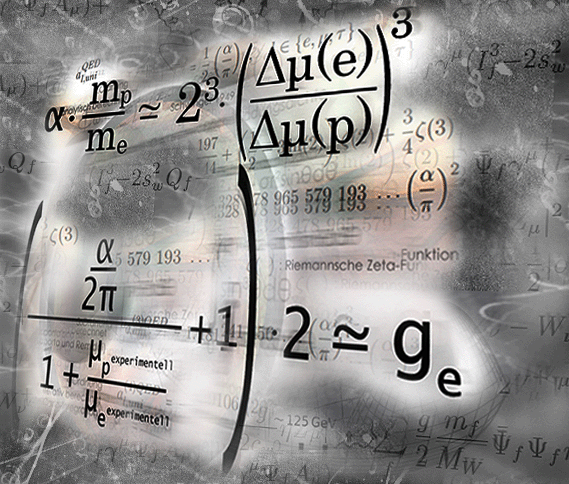Let us continue our analytical „expedition” with a fundamental consideration, which becomes a surprising calculation. One question is: Is the inherent magnetic field contribution to the magnetic moment multi-directional?

If the magnetic field contribution were proportional ("normal") to the mass of the magnetic field interacting charge carrier, the ratio of fe to fp would be equal to the ratio of me to mp.

That's obviously not the case. Measured: 1546.021626754602 = fp / fe = (mp / me) / (μBe / μBp)

Suppose that the magnetic contribution contributes one-dimensionally "abnormally" to the two-dimensional "normal" charge-carrier mass dependence in three-dimensional space. With this assumption we obtain, to a good approximation, for the one-dimensional ("anomalous") part ~ α/8.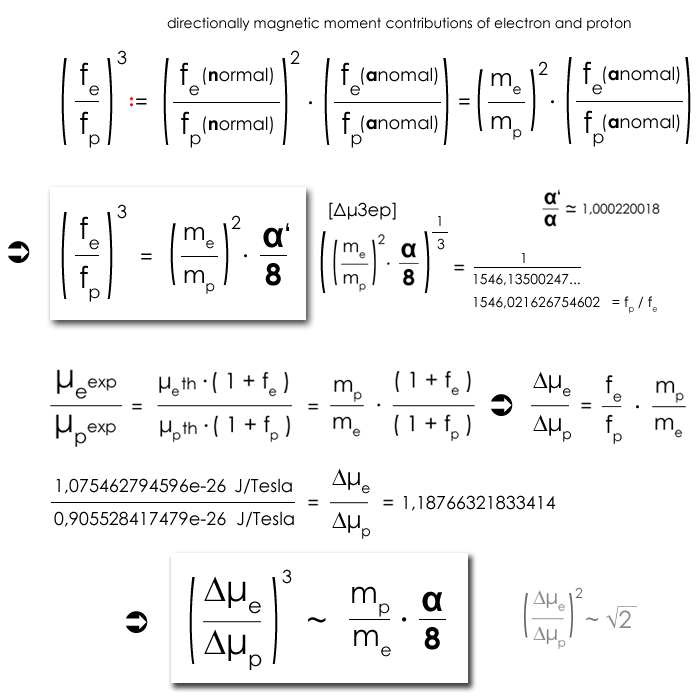details Anatomie anomaler magnetischer Momente.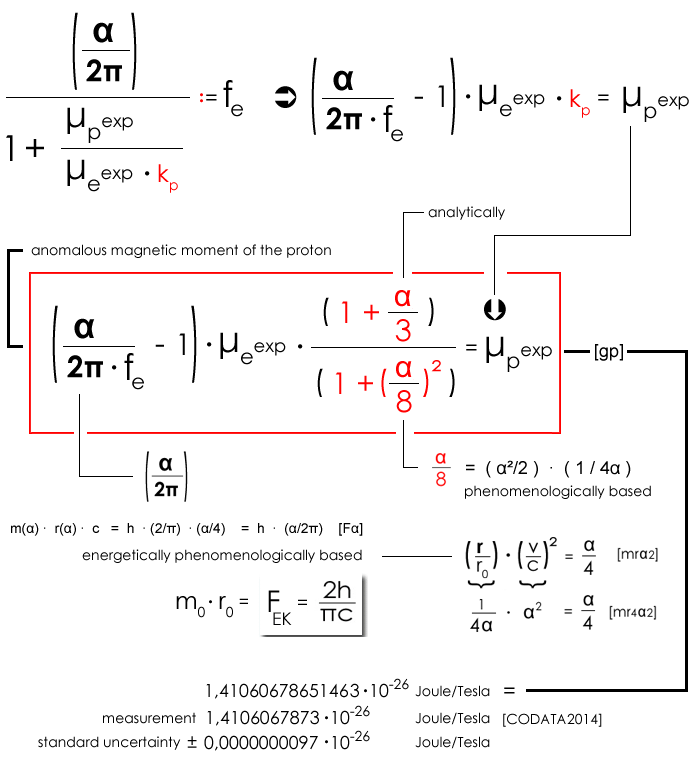Comparing the above parameter-free equation [gp] with the gigantic efforts of standard model physicists to determine the magnetic moment of the proton, shows impressively clear, what plausible reasoned, analytically observational physics is and how extremely minimalistic and expedient the model of a mass-radius coupled space is in connection with an inherent contribution of the external magnetic field to the magnetic moments. Equation [gp] can be easily transformed to the g-factor of the proton :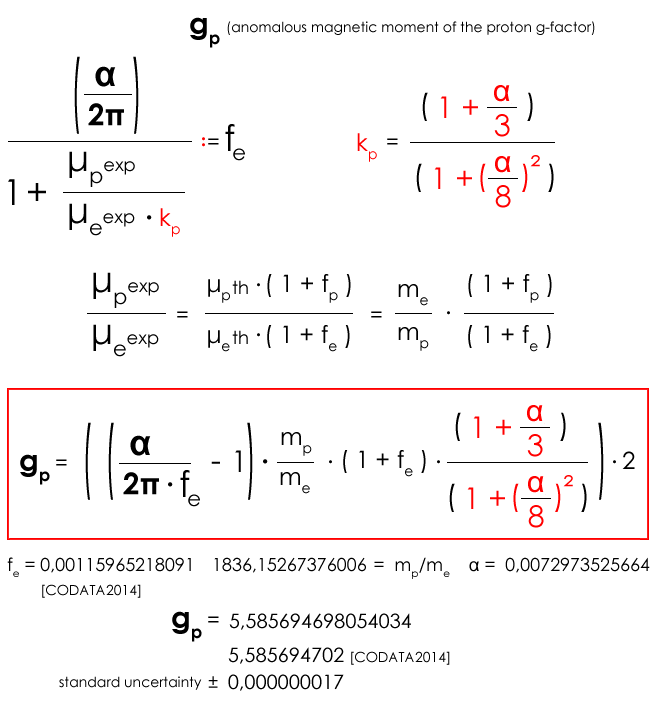electron anomalous magnetic moment calculation

We calculate the electron (anomalous) magnetic moment with "simple" means, that is, based solely on α-terms (... as precisley as it is specified in the "ultra-precise" measurement and QED computer simulations). We start reciprocal-proportional with the same α-terms that were used to (exactly) calculate the g-factor of the proton.details Anatomie anomaler magnetischer Momente.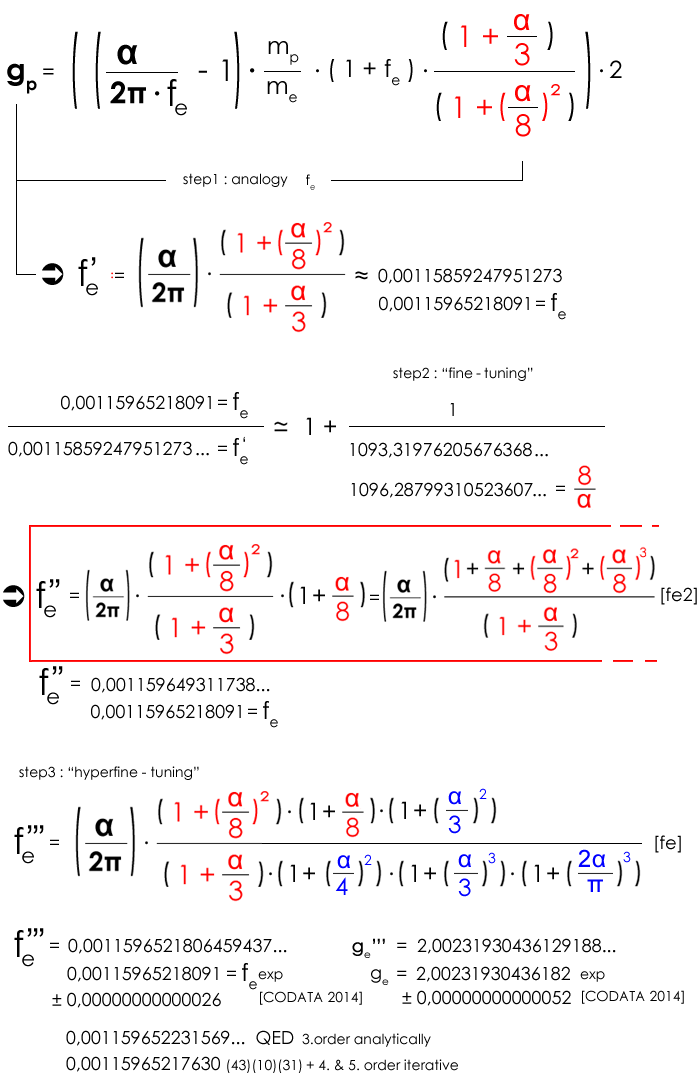For a first orientation, compare the above equations with ~ 13,000 (in words, thirteen thousand !!!) Feynman diagrams and the resulting millions of numerical calculations, of which analytical results are available only up to and including the 3rd order. The (only) analytical QED calculation has a relative standard deviation of only ~ 4.37e-8 rather than the ~ 2.6e-13 (CODATA 2014) to the experimental reading. The "rest" is "faith work" in the form of years of Monte Carlo integrations on computer clusters.

Equation [fe] is a parody of the result-oriented QED "perturbation calculation" (including postulated hadronic contributions) for the determination of the g-factor. For example, the measurement-oriented, numerically-determined "blue terms" could originate from the electric (quadrupole) field of the Penning trap.

The natural-philosophical standards within elementary-body theory allow only »fine-tuning« - expressed by equation [fe2] - to appear consistent and "argumentatively tenable". Thus the magnetic field gives an additive contribution to the magnetic moment of the electron ΔμBe respectively to the fe-value, which depends only on "alpha terms", in very good agreement with the experimental value.

The "red terms" 1+ (α/8) + α/8)˛ + (α/8)3 represent a sequence that could be thought of fractally terms, but mathematically the conceivable supplementary terms (α/8)4, (α/8)5, ..., (α/8)n lay outside the measurable. For even the additive term (α/8)4 increases fe '' only by 0.000000000000101 to 0,00115964931173901 instead of 0,001159649311738. So already clearly outside the specified measuring possibilities.

Present results in the context of a phenomenologically justified mass-radius-coupled magnetic field embodiment stringently followed the numerical analytical conspicuousness of the experimental measurement results and the resulting assumption of measurement-inherent magnetic field contributions. The central "(Schwinger) oscillator term" = (α/2π) is derived from the embodiment of the magnetic field, taking into account the energetic ratios of the electrical energy and the electric elementary charge e compared to the total energy, expressed by the elementary body charge q0. The ratio e/q0 = √α/2 is also consistently decisive for the calculation of the magnetic moment of the neutron from the proton and electron magnetic field contributions ΔμBe and ΔμBp, see equations [μn] and [μn2].

The α-correction calculations for the oscillator term - which lead to fe ('', ''') - are worthy of discussion, since here a (complete) model of the still unknown "(magnetic and electric) field phenomenology" is missing.

In this context, coincidence is phenomenologically (due to the consistency of the model), logically and methodically excluded. The result: Leptonic and quark-based fantasies crumble away. Further consequences: The neutron is electron-proton-based and like the proton without (quarks & Co) substructure. QED has (now) an unsolvable problem; we do not really need to "talk" about QCD here for reasons of insignificance...

to be continued...